# GRE Subject Test: Physics : Length Contraction

## Example Questions

### Example Question #1 : Special Relativity

A meter stick, at an angle of 30 degrees with the x-axis, is traveling at 0.6c in the direction of the positive y-axis.  To a stationary observer, how long does the meter stick appear to be?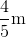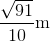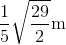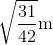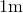Explanation:

Length contraction only occurs in the direction of motion.  This means that the x component of the length, which is cos(30), does not change; length contraction only occurs the the y component, which is sin(30).

First, we find the Lorentz factor: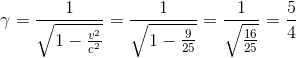Next, we apply the time dilation equation to the length in the y direction: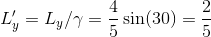Finally, we find the total length by combining the length-contracted y component and the unchanged x component: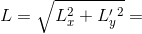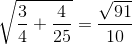### Example Question #1 : Length Contraction

A rocket of length 5 meters passes an observer on earth.  The observer measures the passing rocket to be 3 meters long.  What is the velocity of the rocket in the reference frame of the Earth-based observer?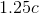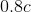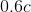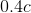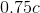Explanation:

Length contraction is given by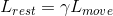Where in this case,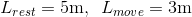The Lorentz factor is given by: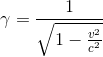Combining these two equations, we get: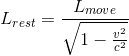Solving for v: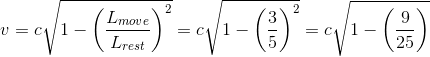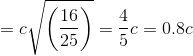### All GRE Subject Test: Physics Resources